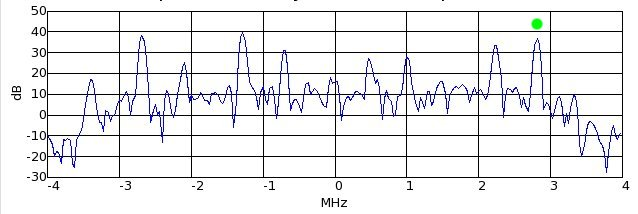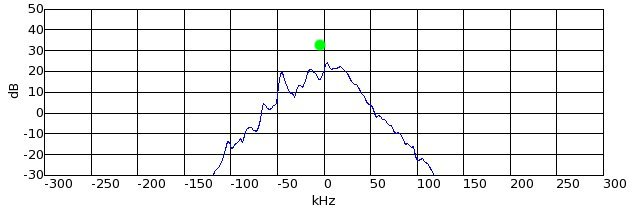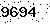FunWithElectronics.com- Collection of Information for those with Electronics as a Hobby
Up one level (GNU Radio and USRP)

## Demodulate FM with GNU Radio

FM capturing consists of three main parts:

1. selecting the correct channel
2. do the actual quadrature demodulation
3. filtering

A typical capture of the FM broadcast band looks like the image below if you put the input into a graphical FFT display. This capture is centered at 100MHz. That means that what is 0MHz on the figure is 100MHz on the air, and what is -2MHz on the figure is 98MHz on the air.#### Step 1 - Choosing the right station

Step 1 is to select the right station. This can be done with the block gr.freq_xlating_fir_filter_ccf. This is a block which combines the process of moving one of the stations to the center and then filter it out from the others.

The station at +2.8MHz marked green, looks like a strong station and lets see how we proceed to get this one out from the others.

```        #!/usr/bin/env python
```

We start off with the common gnuradio initialization.

```	sample_rate = 8000000
decimation = 16
channel_coeffs = gr.firdes.low_pass (1.0, sample_rate, 80e3, 115e3, gr.firdes.WIN_HAMMING)
selectfrequency = gr.freq_xlating_fir_filter_ccf (decimation, channel_coeffs, -2.8e6 , sample_rate)
```

The sample_rate must correspend to the rate the file is captured at. The decimation is the number the sample_rate shall be divided with so that the signal gets a smaller sample rate afterwards, since it's not necessary to have a high sample rate when the signal only contains one channel. The channel_coeffs line is a function which computes some numbers which will be put into the filter, so the filter acts like it should. Here it is specified that it shall be a lowpass filter with a cutoff frequency at 80e3 = 80kHz, and a transition band frequency at 115KHz. The selectfrequency line is where the block to select frequency is written. It takes as input the IF-decimation, the channel_coeffs found on the line above, the frequency shift and the if_rate. Notice that you will need to specify a negative value of -2.8e6 to receive the channel at 2.8e6. You can think of this as like moving the channel -2.8MHz towards the center.

To save the output to a file you can for example use the lines below:

```	fg = gr.top_block()
inputfile = gr.file_source(gr.sizeof_gr_complex,"INPUTFILENAME")
outputfile = gr.file_sink(gr.sizeof_gr_complex,"OUTPUTFILENAME")
fg.connect(inputfile,selectfrequency)
fg.connect(selectfrequency,outputfile)
```

The output after this block will look like below when you feed it into a FFT.Then step 1 is done. Step 1 is common to all kinds of modulations, and is needed everytime the interested signal is not placed exactly surrounding 0Hz.

#### Step 2 - The actual demodulation

Now that we have moved our target station to baseband (centered around 0), it is time to do the actual demodulation. To do this we use the block called gr.quadrature_demod_cf.

```	demod = gr.quadrature_demod_cf(1)
fg.connect(selectfrequency,demod)
```
The output from the demod is actually the audio from the radio channel. This can be fed directly to an audio sink, but normally you would do some filtering first and deemphasizing to get good audio quality. Here I skip the filtering and deemphasizing to make the article simple and short. The audio output is made possible with these functions. The sample rate of the sound is 48000 and the alsa-device is default.
```        audio_out = audio.sink(48000,"default")
fg.connect(demod,audio_out)
```
Finally we start the graph:
```        fg.start()
```Fill in number
Name:
Title:
Text:

tv receiver - sindhuja c (2015-02-19 09:33:50)